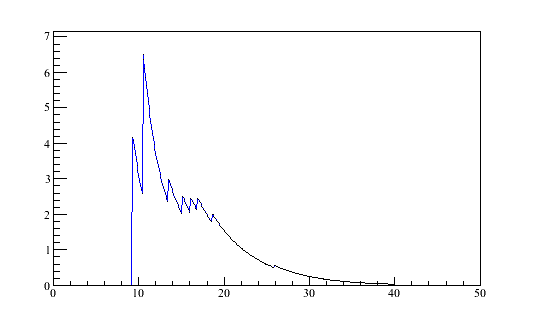# Integral of TGraph

Dear all,

I seem to be misunderstanding the meaning of the TGraph::Integral() method. I would like to call it for the TGraph which I have attached to this post. However, although the graph only contains points with positive or zero y-values,

Graph->Integral()

gives me a negative result. The absolute value of it seems to be reasonable, but with a wrong sign. How should one use this method?

Jenstestgraph.root (9.12 KB)

I have fixed a sign problem in the algorithm showing up with your graph topology.
Could you try with the SVN trunk or wait for our release 5.27/04 tomorrow?

Rene

Hi Rene,

thanks a lot! This works for me, at least in this particular example (I did not try other graphs, though).

Best,

Jens

Hi Rene
Recently the TGraph function was fixed. I would like to call to your attention the boolean limit in the for loop in this function:

``````
Double_t TGraph::Integral(Int_t first, Int_t last) const
{

if (first < 0) first = 0;
if (last < 0) last = fNpoints-1;
if(last >= fNpoints) last = fNpoints-1;
if (first >= last) return 0;
Int_t np = last-first+1;
Double_t sum = 0.0;
//    for(Int_t i=first;i<=last;i++) {
//       Int_t j = first + (i-first+1)%np;
//       sum += TMath::Abs(fX[i]*fY[j]);
//       sum -= TMath::Abs(fY[i]*fX[j]);
//    }
//    return 0.5*sum;

for(Int_t i=first;i<=last;i++) {
Int_t j = first + (i-first+1)%np;
sum += (fY[i]+fY[j])*(fX[j]-fX[i]);
}
return 0.5*TMath::Abs(sum);
}``````

I think the boolean operation should read:

for(Int_t i=first;i<last;i++) { …} i.e. do not include the last index. Consider the case when i=last then the j value will be

Int_t j = first + (last-first+1)%np;
=> Int_t j = first + np%np; i.e replacing by the definition of np== last-first+1
=> j=first
This will produce an error in the value of the integral.